## MP Board Class 8th Maths Solutions Chapter 16 Playing with Numbers Ex 16.1

Find the values of the letters in each of the following and give reasons for the steps involved.
Question 1.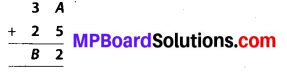Solution:
We have to find the values of A and B. We add the digits column-wise.
In C2 : A + 5 gives 2, that is, a number whose ones digit is 2 i.e., 12.
∴ A + 5 = 12 ⇒ A = 12 – 5 = 7
In C1 : 1 (Carry of C2) + 3 + 2 = B
⇒ 6 = B
∴ We get A = 7, B = 6.

Question 2.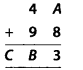Solution:
We have to find the values of A, B and C.
In C3 : A + 8 gives 3, that is, a number whose ones digit is 3 i.e., 13.
Therefore, A + 8 = 13 ⇒ A = 13 – 8 = 5
In C2 : 4 + 9 + 1 (Carry of C3) = 14 gives B = 4
In C1 : 1 (Carry of C2) = 1
Therefore, A = 5, B = 4, C = 1.

Question 3.Solution:
We have to find the value of A.
Since, the ones digit of A × A = A
⇒ A can be 0, 1, 5, 6
If A = 0, then 10 × 0 = 0 ≠ 90
If A = 1, then 11 × 1 = 11 ≠ 91
If A = 5, then 15 × 5 = 75 ≠ 95
If A = 6, then 16 × 6 = 96 = 96
Hence, A = 6.Question 4.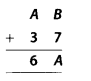Solution:
We have to find the values of A and B.
In C2 : B + 7 – A …………… (i)
In C1 : A + 3 + carry = 6 …………. (ii)
Where, carry is the carry from (i)
Let carry = 0
∴ A + 3 = 6 or A = 3
Putting value of A in (i),
6 + 7 = 3 or 6 = – 4
But B can not be a negative number.
⇒ Carry = 1
In (ii), A + 3 + 1 = 6
⇒ A = 2
Putting value of A in (i), B + 7 = 2
But this is not possible
∴ 6 + 7 = 12 ⇒ 6 = 5.

Question 5.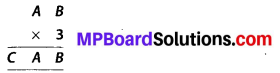Solution:
We have to find the values of A, 6 and C.
Now, looking at ones place
B × 3 = 6
This is possible only when B = 0, 5
Now, writing the equation for multiplication as
3 × (10A + B) = 100C + 10A + B
⇒ 30A + 3B = 100C + 10A + B
⇒ 20A + 2B = 100C
⇒ 50C = 10A + B ………….. (i)
But R.H.S. is the number we multiplied by 3 to obtain CAB.
∴ AB is a multiple of 50.
⇒ B = 0
Equation (i) becomes,
50C = 10A ⇒ 5C = A
Since, A is a single digit number.
∴ C = 1 & A = 5
Hence, A = 5, B = 0, C= 1.

Question 6.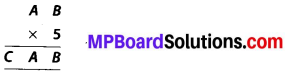Solution:
We have to find the values of A, B and C.
Now, looking at ones place
B × 5 = B
This is possible only when B = 0, 5
Also, writing the equation for multiplication
5 × (10A + B) = 100C + 10A + B
⇒ 50A + 5B = 100C + 10A + B
⇒ 40A + 4B = 100C
⇒ 25C = 10A + B ……………. (i)
But, RHS is the number we multiplied by 5 to obtain result CAB
AB is a multiple of 25.
⇒ B = 0 or 5
(1) Taking B = 0
EQ. (i) becomes
25C = 10A ⇒ 5C = 2A
The only integral one digit solution for this is A = 5, C = 2
(2) Taking B = 5
EQ. (i) becomes
25C = 10A + 5
or 5C = 2A +1
The integral one digit solutions are
A = 2, C = 1
or A = 7, C = 3
Hence, we have three solutions
A = 5, B = 0, C = 2
A = 2, B = 5, C = 1
A = 7, B = 5, C = 3

Question 7.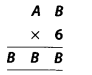Solution:
We have to find the values of A and B.
Writing the multiplication equation
6 × (10A + B) = 100B + 10B + B
⇒ 60A + 6B = 111B
⇒ 60A = 105B
⇒ 4A = 7B
The only integral one digit solution is
A = 7, B = 4Question 8.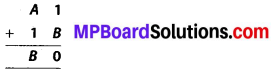Solution:
We have to find the values of A and B. We add the digits column-wise.
In C2 : 1 + B = 10
⇒ B = 9
In C1 : A + 1 + 1 (Carry of C2) = B
⇒ A + 2 = 9
⇒ A = 7
∴ A = 7, B = 9

Question 9.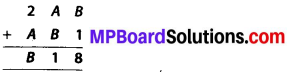Solution:
We have to find the values of A and B.
In C3 : B + 1 = 8
⇒ B = 7
In C2 : A + B = 11 {∵ B is 7 and A can’t be negative}
⇒ A + 7 = 11 ⇒ A = 4
∴ A = 4, B = 7

Question 10.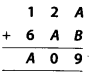Solution:
We have to find the values of A and B.